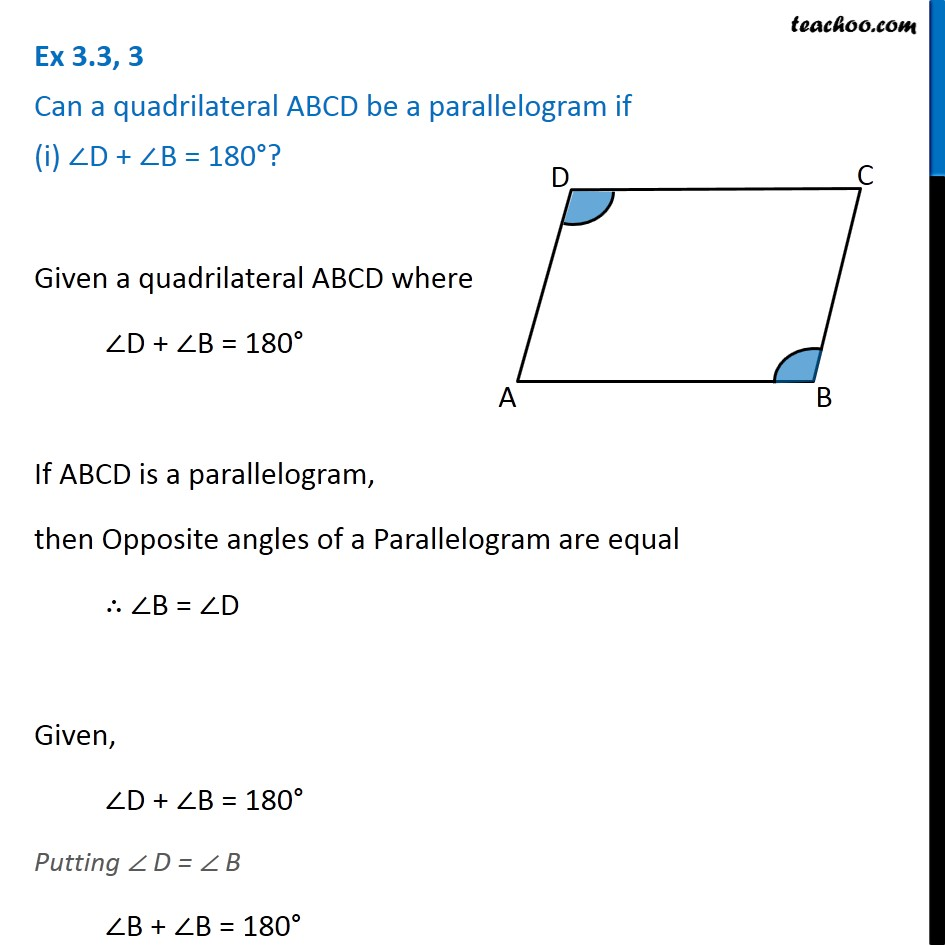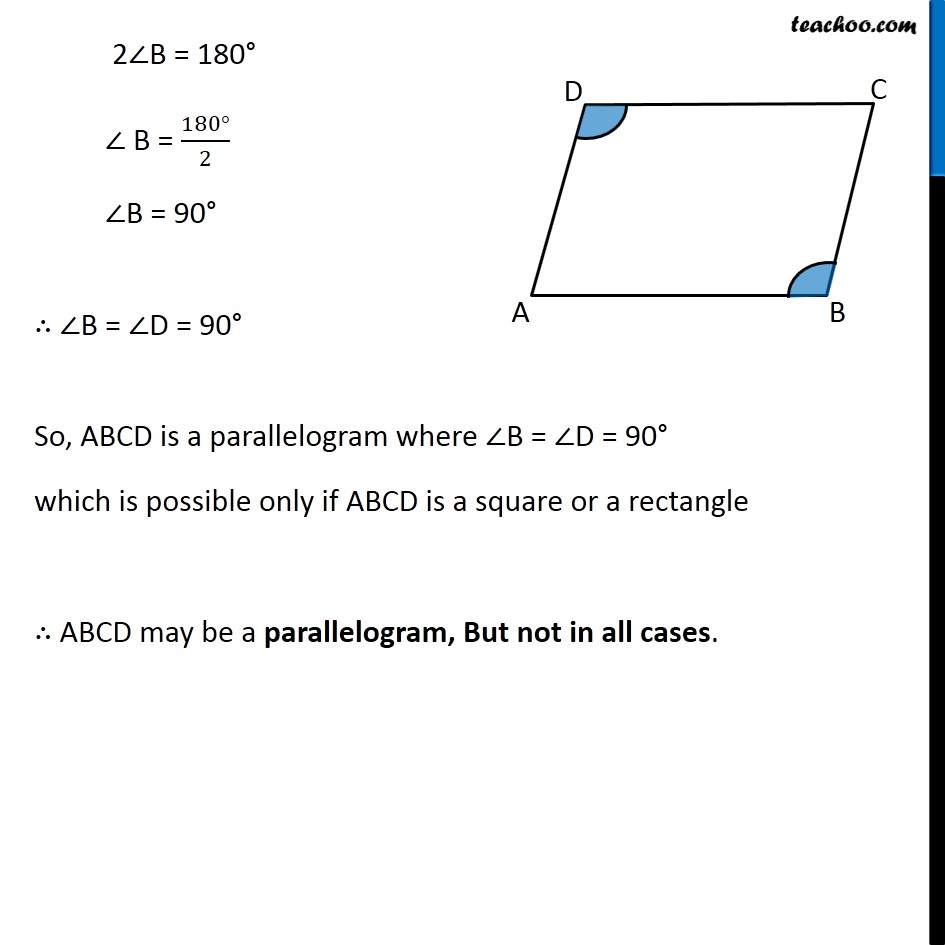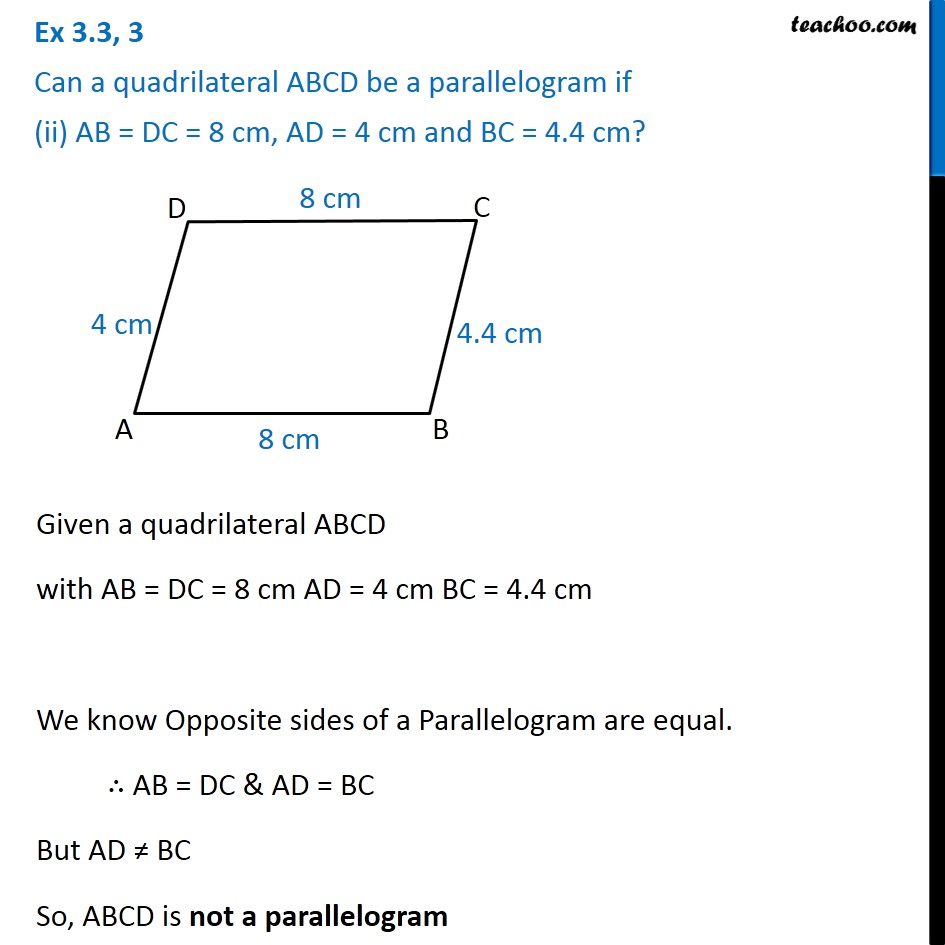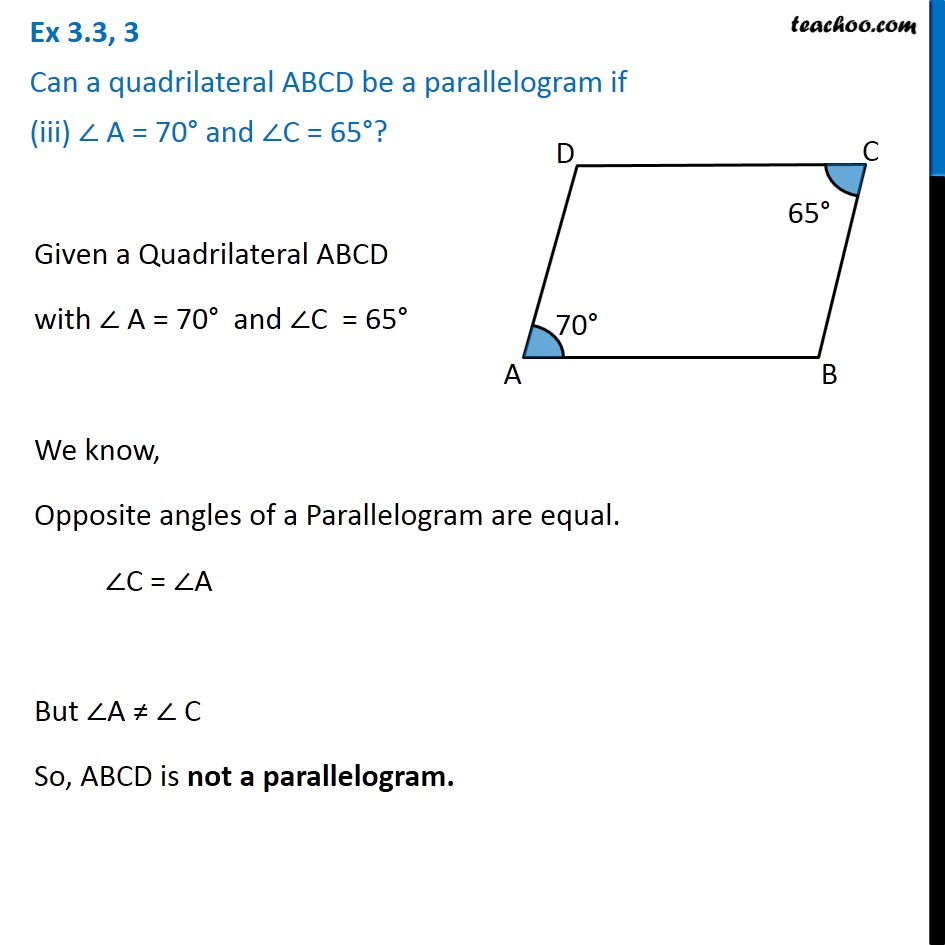Subscribe to our Youtube Channel - https://you.tube/teachoo

1. Chapter 3 Class 8 Understanding Quadrilaterals
2. Serial order wise
3. Ex 3.3

Transcript

Ex 3.3, 3 Can a quadrilateral ABCD be a parallelogram if (i) ∠D + ∠B = 180°?Given a quadrilateral ABCD where ∠D + ∠B = 180° If ABCD is a parallelogram, then Opposite angles of a Parallelogram are equal ∴ ∠B = ∠D Given, ∠D + ∠B = 180° Putting ∠ D = ∠ B ∠B + ∠B = 180° 2∠B = 180° ∠ B = (180°)/2 ∠B = 90° ∴ ∠B = ∠D = 90° So, ABCD is a parallelogram where ∠B = ∠D = 90° which is possible only if ABCD is a square or a rectangle ∴ ABCD may be a parallelogram, But not in all cases. Ex 3.3, 3 Can a quadrilateral ABCD be a parallelogram if (ii) AB = DC = 8 cm, AD = 4 cm and BC = 4.4 cm? Given a quadrilateral ABCD with AB = DC = 8 cm AD = 4 cm BC = 4.4 cm We know Opposite sides of a Parallelogram are equal. ∴ AB = DC & AD = BC But AD ≠ BC So, ABCD is not a parallelogram Ex 3.3, 3 Can a quadrilateral ABCD be a parallelogram if (iii) ∠ A = 70° and ∠C = 65°?Given a Quadrilateral ABCD with ∠ A = 70° and ∠C = 65° We know, Opposite angles of a Parallelogram are equal. ∠C = ∠A But ∠A ≠ ∠ C So, ABCD is not a parallelogram.

Ex 3.3